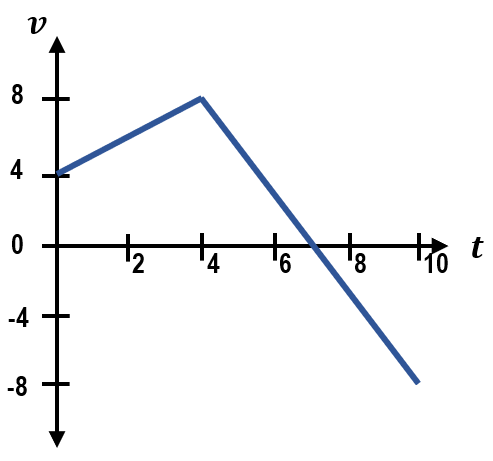## Physics

Learn the toughest concepts covered in Physics with step-by-step video tutorials and practice problems by world-class tutors

2. 1D Motion / Kinematics

# Calculating Displacement from Velocity-Time Graphs

1
concept

## Calculating Displacement from Velocity-Time Graphs4m
Play a video:
2
Problem

A moving box's motion is described by the velocity graph below. The box's initial position is x = 0.
(a) How far has the box moved at t = 4?
(b) What is the displacement of the box from t=4 to t=10?3
example

## Displacement of a Car4m
Play a video: